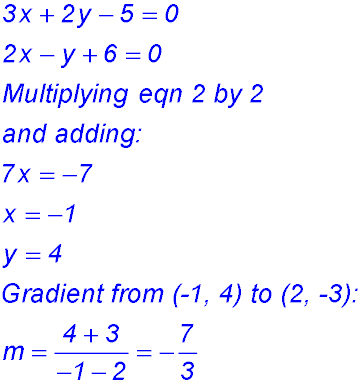Dr. J's Maths.com
Where the techniques of Maths
are explained in simple terms.

Linear functions - Testing for properties of lines.
Test Yourself 1 - Solutions.

 Parallel lines - testing gradient. 1.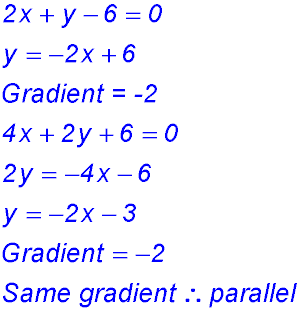2.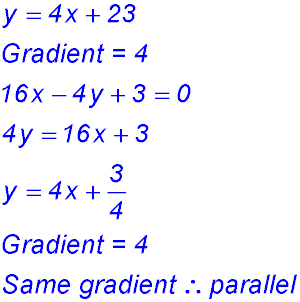3.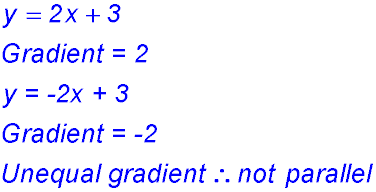4.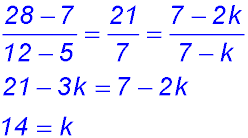5. (i) 3y = x + 1 - gradient is 1/3 (ii) x + 3y + 3 = 0 - gradient is -1/3 (iii) x - 3y = 1 - gradient is 1/3 So (i) and (iii) are parallel. 6.NOTE: it is good practice to calculate all four gradients because if BC // DA, the figure would have been a parallelogram which was not asked. Parallel lines - determining equations. 7.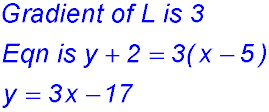8.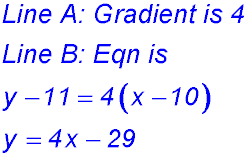9.10.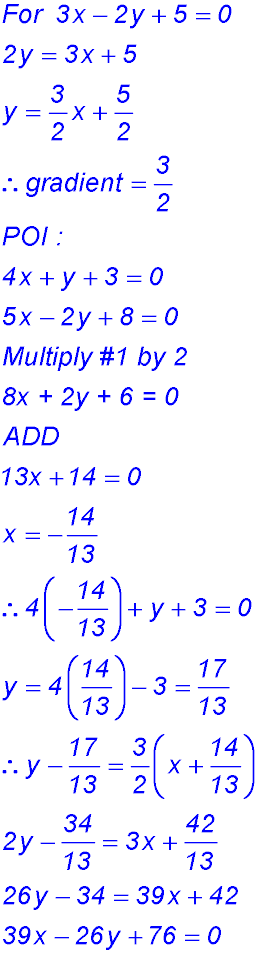11.12. The line joining the points (2, 0) to (0, 4) has gradient of -2 - the rise is 4 and run is 2 and the line slopes down. Hence the tangent to the curve has a gradient of -2. When x = 1 on the parabola y = 1 + 2 = 3. Hence the equation of the tangent to the curve is: y - 3 = -2(x - 1) y = -2x + 5 Perpendicular lines - testing gradients. 13.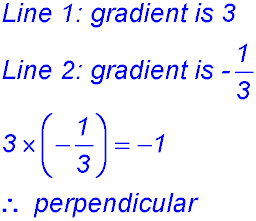14.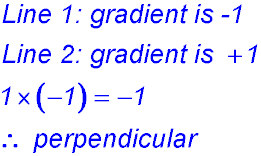15. (i) Gradient is -5. (ii) Gradient is +5. (iii) Gradient is -5. (iv) Gradient is -15. (v) Gradient is +5 Hence lines (i) and (iii) are parallel. Lines (ii) and (v) are parallel. 16.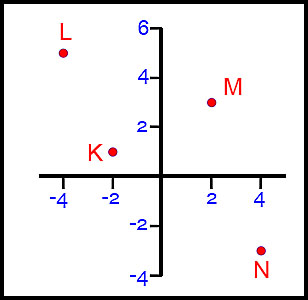(ii)(iii)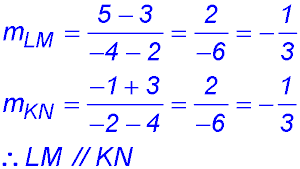(iv)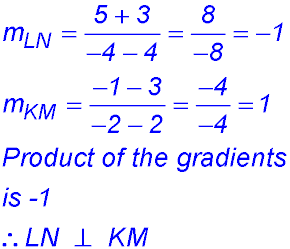(v) The quadrilateral has opposite sides parallel. So it is a parallelogram at least. KL is not perpendicular to LM so the figure is not a rectangle. The diagonals cut each other at right angles - so the parallelogram can be refined to a rhombus. See the Quadrilateral Family. 17. 18.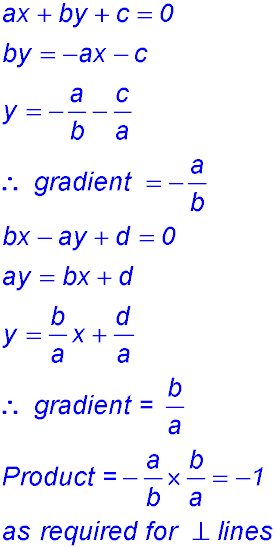Perpendicular lines - determining equations. 19.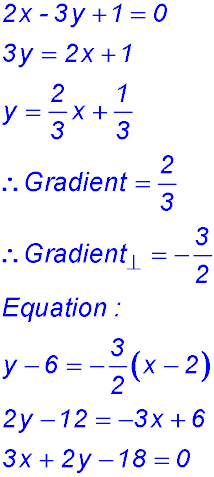20.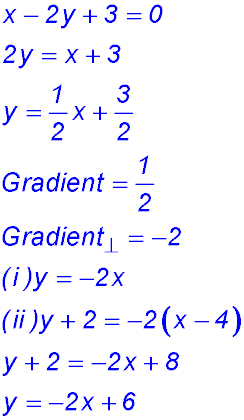21.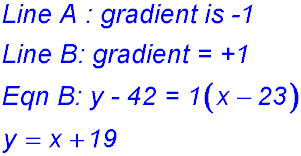22.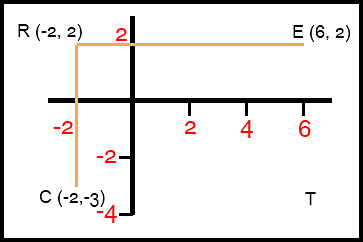(ii) CT is a horizontal line parallel to RE and passing through y = -3. Hence CT is y = -3 ET is a vertical line parallel to RC and passing through x = 6. Hence ET is x = 6. 23. 24. Collinear points. 25.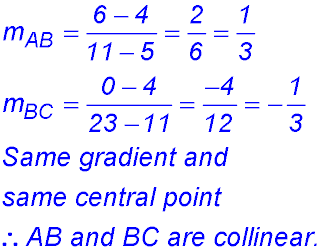26.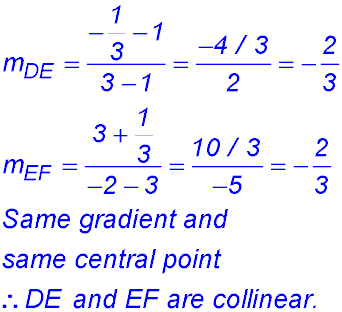27.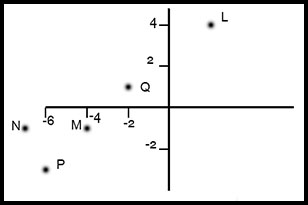(i) The sketch gives a hint that the points could be M, P and Q. Calculate the gradients between these points: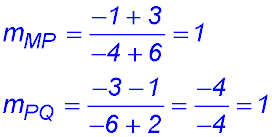Same gradient in each direction and point P links to both M and Q. ∴ M, P and Q are collinear. (ii) Link the new point to any of the three and calculate the gradient: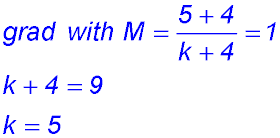28. (i)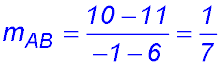(ii)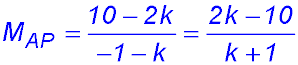(iii)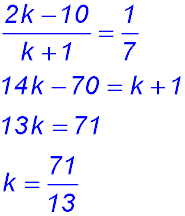29. Calculate the gradient AB and then set this value against the gradients for AC and then AD: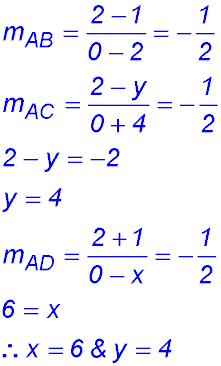30.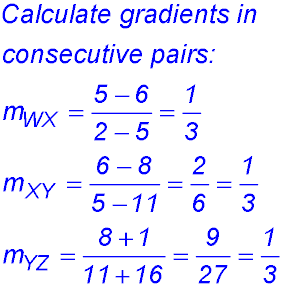All gradients are the same and each pair is linked. Hence the four points are collinear. Concurrent lines. 31. Eqn (2) - Eqn (1): 4y = 8 y = 2 Substitute into Eqn (2): 2x = 0 x = 0 Sub. x = 0 and y = 2 into Eqn (3): LHS = 2 RHS = 10(0) + 2 = 2 = LHS. ∴ all 3 lines passs through the same point. Hence they are concurrent. 32. (i) Point of intersection between the lines x + 2y - 6 = 0 and 3x - 2y - 6 = 0. Add the 2 eqns: 4x - 12 = 0 x = 3 Substituting into eqn 1: 2y = 3 y = 1.5 POI is (3, 1.5) (ii) To be concurrent, the new line must pass through the POI: ∴ k(3) + 3(1.5) - 6 = 0 3k = 1.5 k = 0.5. 33. Add Eqn (1) and Eqn (2): 3x = 3 x = 1 Sub into Eqn (1): 1 + y - 2 = 0 y = 1. Sub (1, 1) into Eqn (3): LHS = 1 + 3 - 4 = 0 = RHS ∴ all 3 lines passs through the same point. Hence they are concurrent. 34. 35. (i)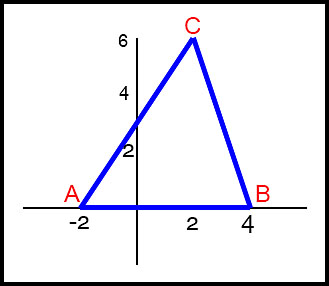(ii)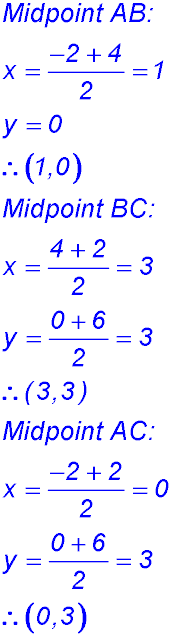(iii) Determine the equation of the line perpendicular to each side and passing through the midpoint of each side. AB: Midpoint is (1, 0) and gradient is 0 (horizontal. Hence vertical bisector is x = 1. BC: Midpoint is (3, 3) and gradient is -3. Eqn of perpendicular: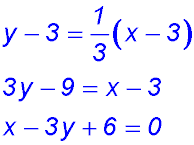AC: Midpoint is (0, 3) and gradient is 1.5. Eqn of perpendicular: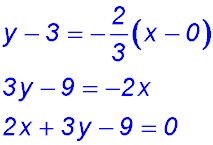(iv) Substitute x = 1 into Eqn BC bisector: y = 7/3 Substitute x = 1 into Eqn AC bisector: y = 7/3 All lines meet at the same point so they are concurrent. 36. If concurrent, the two given equations must be solved simultaneously: